# How to Sum If Date is Greater or Less Than in Excel

If we sum for certain conditions, we’ll use the SUMIF function in excel. For example, count the total sales for a period before of after a specified date. This article will help you to do sum for a specified period by SUMIF function.

## SUMIF Date is Less/Greater than Specified Date in Excel

Prepare a table with dates and sales.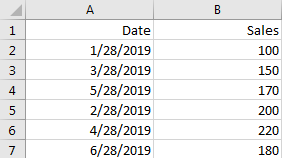If we want to count the total sales for dates before a specified date, we can follow below steps to do this.

Step 1: Prepare another table to save the specified date and total sales.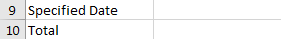Step 2: type the Specified Date in Cell. For example: 5/28/2019; then enter the below formula based on the SUMIF function:

`=SUMIF(A2:A7,"<"&B9,B2:B7)`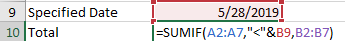In above formula, A2:A7 is the range of all dates, B9 is the specified date, B2:B7 is the range of sales. You can change the date and range in the formula based on your needs.

Step 3: Click Enter to get the result.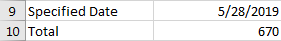If you want to count the total for sales greater than a specified date and includes that date, you can use below formula.

Step 4: In B10, enter the below formula:

`=SUMIF(A2:A7,">="&B9,B2:B7)`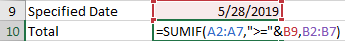Step 5: Click Enter to get the result.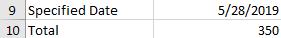Note:

If you want count the total sales before today, you can directly enter the following formula based on the SUMIF function and the TODAY function:

`=SUMIF(A2:A7,"<"&TODAY(),B2:B7)`

### Related Functions

• Excel TODAY function
The Excel TODAY function returns the serial number of the current date. So you can get the current system date from the TODAY function. The syntax of the TODAY function is as below:=TODAY()…
• Excel SUMIF Function
The Excel SUMIF function sum the numbers in the range of cells that meet a single criteria that you specify. The syntax of the SUMIF function is as below:=SUMIF (range, criteria, [sum_range])…

Related Posts

Calculate Days Open in Excel

If you want to know how to Calculate days in Excel, there are some formulas that you can use to do so. For example, you can use the DAYS function in Excel to find the number of days between two ...

How to Sum Last N Days in Excel

In our daily life, we may want to sum amounts or sales for a specific period, for example in last N days. Sum numbers in Excel is easy to run, we can apply SUM function. But if we want to ...

How to Sum Numbers by Formula if Cells Are Equal to A Certain Value in Excel

We may meet the case that sum numbers based on a certain value in daily work. To sum numbers based on a certain value, we can define this value as a criterion. Then sum up all numbers which meet the ...

How to Sum Data if Begins with/End with/Contains in Excel

For a set of data, sometimes we may need to sum some of them based on criteria ‘if begins with/end with/contains’ ** characters. In our daily work, this case frequently occurs when calculating total for one or more items among ...

How to Sum by Group in Excel

Sometimes we may have the requirement that subtotal data by group in a table. To just subtotal data in a column, we can use SUM function directly; for data in a column, amount data in another column, we can use ...

How to Subtotal for Value in Specific Cell in Excel

Sometimes we may want to sum within some criteria in excel, actually, we can use SUMIF function directly instead of SUM for some special instance. For example, if we just want to get subtotal for one product among all products, ...

How to Sum Values Based on Selection of Drop-Down List in Excel?

We can do SUM for a list or a range of values easily if we select all values together and then apply SUM function in excel. But if we only want to sum part of them based on the selection ...

How to Calculate Retirement Date and Remaining Years refer to Birth Date in Excel

Every company has its own police about the retirement date for employees. Suppose employees will be retired from a certain age of 60, how can we calculate the retirement date for everyone base on their birthdays? Actually, there are several ...

How to Highlight Cell or Row If Date Is In Current Day/Week/Month in Excel

This post will guide you how to highlight cell if date is the current day or is in the current week or month in Excel. How do I highlight row if date is in current week or month with conditional ...

How to Add the Current Month or Year in a Cell in Excel

This post will guide you how to add the current month or year into a cell or header or footer in your worksheet. How do I insert the current date or time in a cell with a formula in Excel. ...

Sidebar